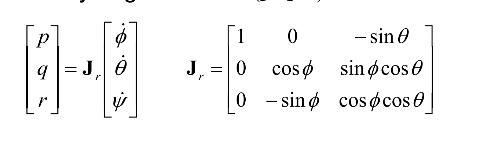# Fixed-wing Attitude Controller for VTOL Aircraft

Hi everyone,

I have some questions on the Fixed-wing Attitude Controller.

Question 1:
Where could I find P in a script and the parameter definition in https://dev.px4.io/v1.9.0/en/advanced/parameter_reference.html?

Question 2:
What are the equations in the “Turn coordination block”?

Question 3:
Could somebody spell out the Jacobian Matrix?

Hi, here you go:

1. The P controller has separate gains for Roll and Pitch, and they are actually inverted (time constants): FW_R_TC and FW_P_TC

2. It’s about how much the vehicle need to yaw to achieve a coordinated turn. This is the most common formula thereIn PX4 there is also taken the pitch into account for this, see https://github.com/PX4/ecl/blob/9b4b24ee71902c6a4289170e8fb1ca3306c1d9c4/attitude_fw/ecl_yaw_controller.cpp#L105

3.Feel free to ask if stuff is still unclear!

Thank you very much for your response.
I have another question about the Fixed-Wing Mixer block in the diagram above.
I’m trying to find the equations used in the Mixer block. Would you be able to spell out the equations in the block?

See here. For a Fw vehicle the mixer is quite straight forward, eg
elevator_deflection = trim + pitching_rate_sp * scale

Thank you very much for your help.
I’m looking for the codes for the expression below in a script.
elevator_deflection = trim + pitching_rate_sp * scale
Could you point out the location of the codes?

This is the mixer for fixed-wing vehicles (it’s called simple mixer because all axis are decoupled, not because the code itself is very simple ^^)

And this is en example of a configuration file for a standard FW vehicle:

Thank you very much for your help. I appreciate it.

Hello, I have another question about the yaw stick command below for stabilized mode.

In the diagram below, where is the yaw stick command (yaw rate) added? After the “Turn coordination” block?

And, where in a script could I find the yaw stick command addition?

@crest_wave not quite, the yaw stick command is added after the rate controller additionally to what the controller sets: https://github.com/PX4/Firmware/blob/f0dde453032b3bf40e7c7f90e766054f385d5258/src/modules/fw_att_control/FixedwingAttitudeControl.cpp#L554.

In the script below, it says “/* add in manual rudder control in manual modes */”.
So, does it also apply to stabilized mode?

I have another question for multicopter stabilized mode in the post below.
If you are familiar with the issue and can answer to my question, that would be much appreciated.

Manual modes are all modes are all modes in which the user interacts with a RC/joystick, so full manual, stabilized, altitude control, position control.

1 Like

Thank you very much for your help. I got it!

Hello! I am confused that why there is need to add a Jacobian Matrix here? because the phi, thita and ps are Euler angles and their dericatives are attitude rate.

Thank you very much!

because the phi, thita and ps are Euler angles and their dericatives are attitude rate

Not directly, body rates are not the same as attitude derivatives. That’s only correct for 0 roll and pitch (in which case the Jacobian becomes an identity matrix).

Hello! I have some questions related to mixer and servo modules.

1. In the user guide, in order to explain how mixer works, an usage example is developed and the output is this:

My questions are about the variables that appear in this expression:
• I imagine that SERVO will be the input in servo block, so is it the pulse width expressed in ms?
• Taking into account the FW Attitude Controller diagram, the mixer input is the derivative of body rate vector, so are the pitch/roll inputs angles or are they angular accelerations?
1. If u is the pulse width, where could I find how the servo block works to get the angular deflection of control surface?